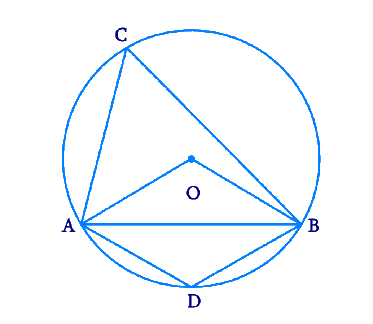In the verge of coronavirus pandemic, we are providing FREE access to our entire Online Curriculum to ensure Learning Doesn't STOP!

# Ex.10.5 Q2 Circles Solution - NCERT Maths Class 9

Go back to  'Ex.10.5'

## Question

A chord of a circle is equal to the radius of the circle. Find the angle subtended by the chord at a point on the minor arc and also at a point on the major arc.

Video Solution
Circles
Ex 10.5 | Question 2

## Text Solution

What is known?

Chord’s length is equal to the radius.

What is unknown?

Angle subtended by the chord at a point on the minor arc and also at a point on the major arc.

Reasoning:

• The angle subtended by an arc at the centre is double the angle subtended by it at any point on the remaining part of the circle.
• A quadrilateral $$ABCD$$ is called cyclic if all the four vertices of it lie on a circle.
• The sum of either pair of opposite angles of a cyclic quadrilateral is \begin{align}180^{\circ}.\end {align}

Steps:Draw a circle with any radius and centre $$O$$. Let $$AO$$ and $$BO$$ be the $$2$$ radii of the circle and let $$AB$$ be the chord equal to the length of radius. Join them to form a triangle.

$$OA = OB = AB$$

Hence \begin{align} \Delta {ABO} \end {align} becomes an equilateral triangle.

Draw $$2$$ points $$C$$ and $$D$$ on the circle such that they lie on major arc and minor arc respectively.

Since \begin{align} \Delta {ABO} \end {align} is an equilateral triangle, we get \begin{align} \angle {ABO}=60^{\circ} \end {align}

For the arc $$AB,$$ \begin {align} \angle {AOB}=2 \angle {ACB} \end {align} as we know that the angle subtended by an arc at the centre is double the angle subtended by it at any point on the remaining part of the circle.

\begin{align}\angle ACB &= \frac{1}{2}\angle AOB\\&= \frac{1}{2} \times 60 \\&= 30^\circ \end{align}

As you can notice the points $$A, B, C$$ and $$D$$ lie on the circle. Hence $$A B C D$$ is a cyclic quadrilateral.

We know that, the sum of either pair of opposite angles of a cyclic quadrilateral is \begin{align}180^\circ.\end {align}

Therefore,

\begin{align}\angle ACB + \angle ADB &= 180^\circ \\30 + \angle ADB &= 180\\\angle ADB &= 150^\circ \end{align}

So when the chord of a circle is equal to the radius of the circle, the angle subtended by the chord at a point on the minor arc is \begin{align}150^\circ.\end {align} and also at a point on the major arc is \begin{align}30^\circ.\end {align}

Video Solution
Circles
Ex 10.5 | Question 2

Learn from the best math teachers and top your exams

• Live one on one classroom and doubt clearing
• Practice worksheets in and after class for conceptual clarity
• Personalized curriculum to keep up with school